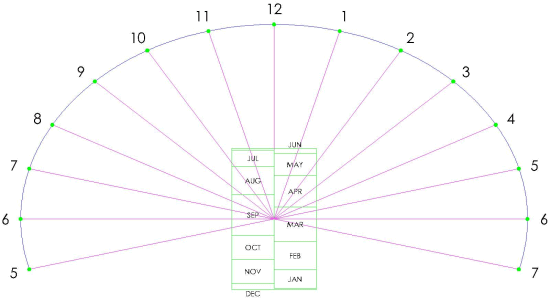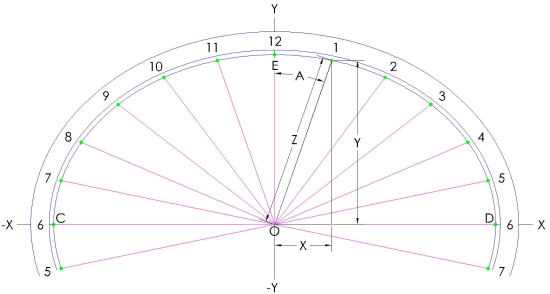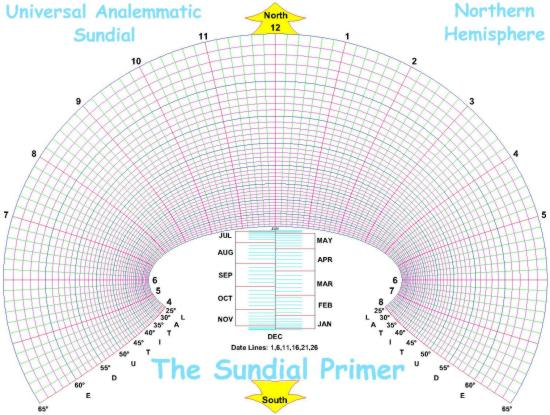The Sundial Primer created by Carl SabanskiThe Sundial Primer Index
 Universal Analemmatic Sundial Analemmatic Sundial: sundials consisting of hour points (rather than hour lines) laid round an ellipse, and a movable gnomon perpendicular to the dial plane. It may be on any plane, but the most usual form is horizontal. In the horizontal version of the dial, gnomon position lies on the straight North-South minor axis, at a point determined by the sun's declination (i.e. the date). The analemmatic dial may be regarded as a projection of the universal equatorial ring dial.Declination (of the Sun): the angular distance of the Sun above or below the celestial equator. Its value follows an annual sine wave like curve, varying between 0° at the equinoxes and ±23.4° (approx.) at the solstices. It has positive values when the Sun is above the celestial equator (summer in the Northern hemisphere) and negative when below. Figure 1 illustrates a typical analemmatic sundial with its scale of dates.Figure 1: Analemmatic Sundial (CAD) Figure 2 illustrates the design layout for the sundial and the following equations are used to calculate the X and Y co-ordinates of the hour points and the points for the scale of dates.Figure 2: Analemmatic Sundial Layout (CAD) The horizontal dimension "X" from point "O" is:X = sin h where h is the hour angle, in degrees, given by:h = (T24 - 12) * 15°and T24 is the time in 24-hour clock notation (hours after midnight) in decimal hours.The vertical dimension "Y" from point "O" is:Y = sin ø * cos hNote that the calculation of "X" only requires the hour angle and would be the same for a dial at any latitude. The dimension "Y" is dependent upon the latitude.The next step is to establish the scale of dates. This is the scale that is used to determine the position of the gnomon on a given day of the year. The scale points can be determined for any day of the month but typically the first day is used and the summer and winter solstices establish the extremes of the scale. The scale of dates is determined as follows:S = tan (dec) * cos øwhere "dec" is the sun's declination.For more details check out the "Analemmatic Sundial" page.It is possible to manipulate the equations above to create a universal analemmatic sundial. To do this the equation for the scale of dates must be made independent of the latitude "ø".  This is done by dividing all three equations by the term "cos ø". As a result, the equations become:X = sin h / cos ø Y = tan ø * cos hS = tan (dec)The scale of dates is now dependent upon only the sun's declination for any latitude. The X and Y co-ordinates can be calculated for any number of hour points at any latitude.Figure 3 illustrates the layout of a universal analemmatic sundial. Although it may appear there are hour lines, the time is determined by the point the gnomon's shadow falls on for a particular latitude ellipse. This sundial will operate in the latitude range of 25° to 65° in the Northern Hemisphere. For the Southern Hemisphere the hour numbers will be reversed and the scale of dates will be a mirror image about the semi-major axis. The "North" and "South" arrows will be reversed.Figure 3: Universal Analemmatic Sundial Layout (CAD)